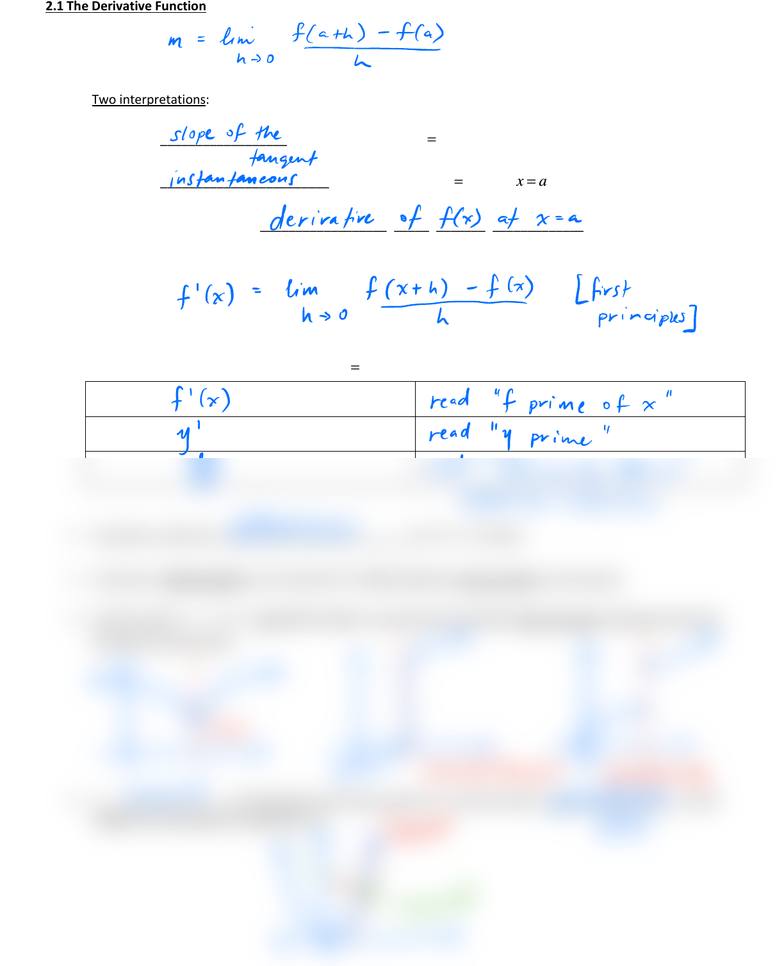# MAT135H1 Lecture Notes - Lecture 2: Ath

19 views2 pages2.1 The Derivative Function
recall:
Two interpretations:
1) the _________________________ to the graph
()
yfx
=
at point
(, ())
afa
2) the _________________________ rate of change of
()
yfx
=
at
xa
=
In calculus, this is called the _____________________________________________________
In general, the derivative function for any function
()
fx
is given by:
Notation for the derivative of the function
()
yfx
=
a function is said to be __________________________ at a if
'( )
fa
exists
a function is differentiable on an interval if it is differentiable at every number in the interval
at points where
()
yfx
=
is not differentiable, we say that the derivative does not exist; examples where the
derivative does not exist:
a __________________ to the graph of a function at point P, is the line that is ___________________ to the
tangent line that passes through point P.
mlenniofath f
h
slopeof the
tangent
instantaneous
fxhim fCxth
hfirst
h0principles
Leibniz notation
differentiable
disdontinuity
normal i
Unlock document

This preview shows half of the first page of the document.
Unlock all 2 pages and 3 million more documents.